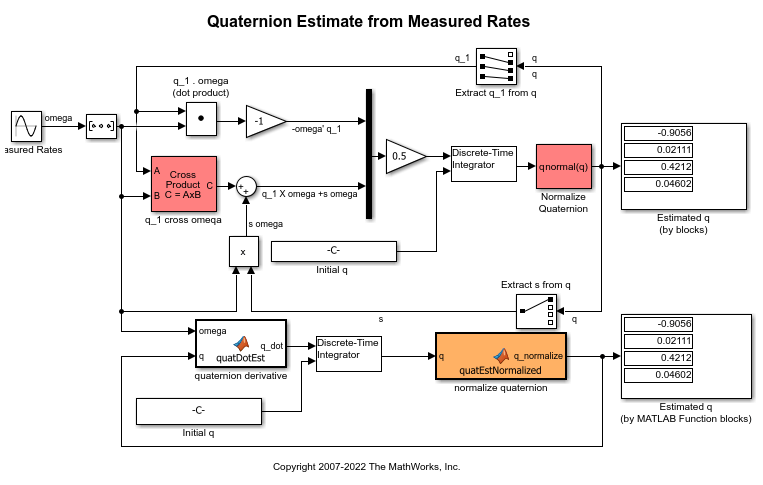# Quaternion Estimate from Measured Rates

This example shows how to estimate a quaternion and model the equations in the following ways:

• Using Simulink® and Aerospace Blockset™ software to implement the equations.

• Using MATLAB® Function block to incorporate an Aerospace Toolbox quaternion function.

This model has been color coded to aid in locating Aerospace Blockset blocks.

The red blocks are Aerospace Blockset blocks, the orange block is a MATLAB Function block containing a function with MATLAB function block support provided by Aerospace Blockset and the white blocks are Simulink blocks.